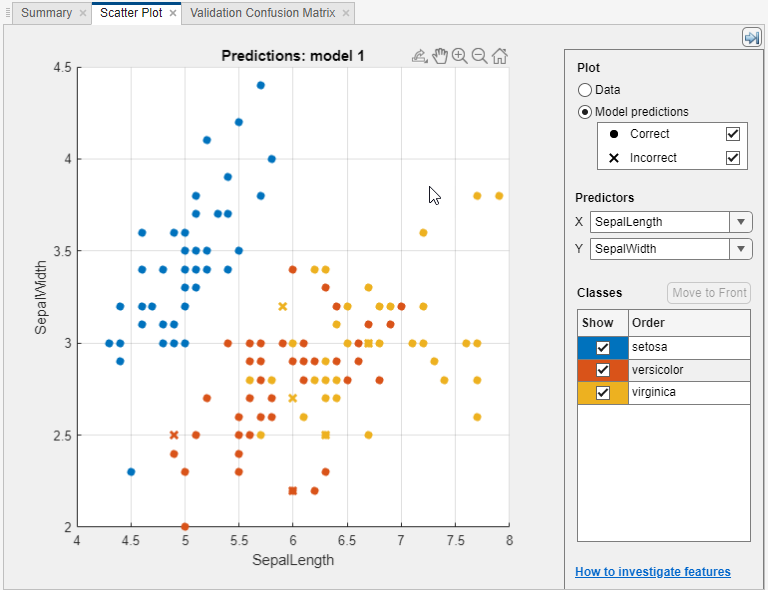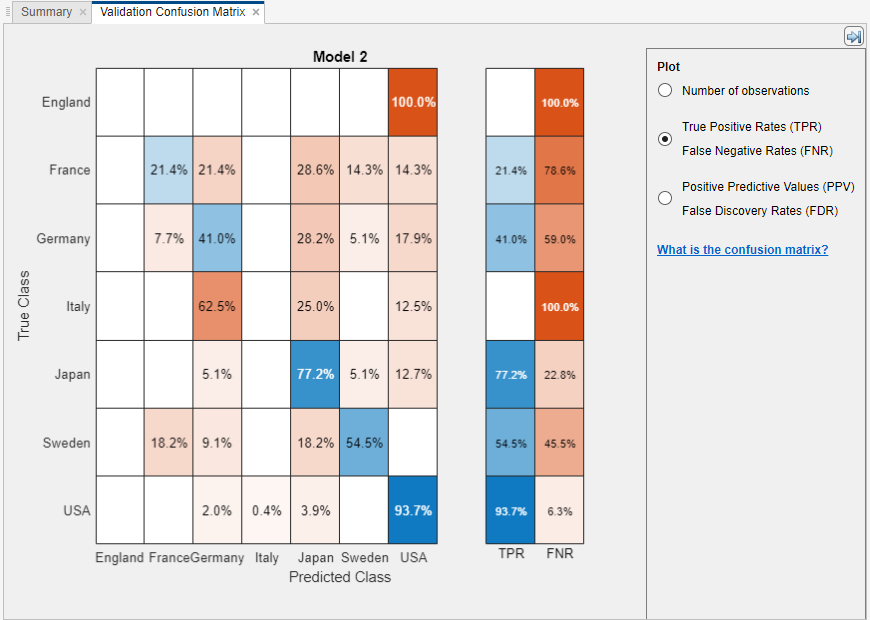## Assess Classifier Performance in Classification Learner

After training classifiers in Classification Learner, you can compare models based on accuracy scores, visualize results by plotting class predictions, and check performance using confusion matrix and ROC curve.

• If you use k-fold cross-validation, then the app computes the accuracy scores using the observations in the k validation folds and reports the average cross-validation error. It also makes predictions on the observations in these validation folds and computes the confusion matrix and ROC curve based on these predictions.

### Note

When you import data into the app, if you accept the defaults, the app automatically uses cross-validation. To learn more, see Choose Validation Scheme.

• If you use hold-out validation, the app computes the accuracy scores using the observations in the validation fold and makes predictions on these observations. The app also computes the confusion matrix and ROC curve based on these predictions.

• If you choose not to use a validation scheme, the score is the resubstitution accuracy based on all the training data, and the predictions are resubstitution predictions.

### Check Performance in the History List

After training a model in Classification Learner, check the History list to see which model has the best overall accuracy in percent. The best Accuracy score is highlighted in a box. This score is the validation accuracy (unless you opted for no validation scheme). The validation accuracy score estimates a model's performance on new data compared to the training data. Use the score to help you choose the best model.

• For cross-validation, the score is the accuracy on all observations, counting each observation when it was in a held-out fold.

• For holdout validation, the score is the accuracy on the held-out observations.

• For no validation, the score is the resubstitution accuracy against all the training data observations.

The best overall score might not be the best model for your goal. A model with a slightly lower overall accuracy might be the best classifier for your goal. For example, false positives in a particular class might be important to you. You might want to exclude some predictors where data collection is expensive or difficult.

To find out how the classifier performed in each class, examine the confusion matrix.

### Plot Classifier Results

In the scatter plot, view the classifier results. After you train a classifier, the scatter plot switches from displaying the data to showing model predictions. If you are using holdout or cross-validation, then these predictions are the predictions on the held-out observations. In other words, each prediction is obtained using a model that was trained without using the corresponding observation. To investigate your results, use the controls on the right. You can:

• Choose whether to plot model predictions or the data alone.

• Show or hide correct or incorrect results using the check boxes under Model predictions.

• Choose features to plot using the X and Y lists under Predictors.

• Visualize results by class by showing or hiding specific classes using the check boxes under Show.

• Change the stacking order of the plotted classes by selecting a class under Classes and then clicking Move to Front.

• Zoom in and out, or pan across the plot. To enable zooming or panning, hover the mouse over the scatter plot and click the corresponding button on the toolbar that appears above the top right of the plot.To export the scatter plots you create in the app to figures, see Export Plots in Classification Learner App.

### Check Performance Per Class in the Confusion Matrix

Use the confusion matrix plot to understand how the currently selected classifier performed in each class. To view the confusion matrix after training a model, click Confusion Matrix in the Plots section of the Classification Learner tab. The confusion matrix helps you identify the areas where the classifier has performed poorly.

When you open the plot, the rows show the true class, and the columns show the predicted class. If you are using holdout or cross-validation, then the confusion matrix is calculated using the predictions on the held-out observations. The diagonal cells show where the true class and predicted class match. If these diagonal cells are blue, the classifier has classified observations of this true class are classified correctly.

The default view shows the number of observations in each cell.

To see how the classifier performed per class, under Plot, select the True Positive Rates (TPR), False Negative Rates (FNR) option. The TPR is the proportion of correctly classified observations per true class. The FNR is the proportion of incorrectly classified observations per true class. The plot shows summaries per true class in the last two columns on the right.

### Tip

Look for areas where the classifier performed poorly by examining cells off the diagonal that display high percentages and are orange. The higher the percentage, the darker the hue of the cell color. In these orange cells, the true class and the predicted class do not match. The data points are misclassified.In this example, which uses the `carsmall` data set, the top row shows all cars with the true class France. The columns show the predicted classes. In the top row, 25% of the cars from France are correctly classified, so 25% is the true positive rate for correctly classified points in this class, shown in the blue cell in the TPR column.

The other cars in the France row are misclassified: 25% of the cars are incorrectly classified as from Japan, and 50% are classified as from USA. The false negative rate for incorrectly classified points in this class is 75%, shown in the orange cell in the FNR column.

If you want to see numbers of observations (cars, in this example) instead of percentages, under Plot, select Number of observations.

If false positives are important in your classification problem, plot results per predicted class (instead of true class) to investigate false discovery rates. To see results per predicted class, under Plot, select the Positive Predictive Values (PPV), False Discovery Rates (FDR) option. The PPV is the proportion of correctly classified observations per predicted class. The FDR is the proportion of incorrectly classified observations per predicted class. With this option selected, the confusion matrix now includes summary rows below the table. Positive predictive values are shown in blue for the correctly predicted points in each class, and false discovery rates are shown in orange for the incorrectly predicted points in each class.

If you decide there are too many misclassified points in the classes of interest, try changing classifier settings or feature selection to search for a better model.

To export the confusion matrix plots you create in the app to figures, see Export Plots in Classification Learner App.

### Check the ROC Curve

To view the ROC curve after training a model, on the Classification Learner tab, in the Plots section, click ROC Curve. View the receiver operating characteristic (ROC) curve showing true and false positive rates. The ROC curve shows true positive rate versus false positive rate for the currently selected trained classifier. You can select different classes to plot.

The marker on the plot shows the performance of the currently selected classifier. The marker shows the values of the false positive rate (FPR) and the true positive rate (TPR) for the currently selected classifier. For example, a false positive rate (FPR) of 0.2 indicates that the current classifier assigns 20% of the observations incorrectly to the positive class. A true positive rate of 0.9 indicates that the current classifier assigns 90% of the observations correctly to the positive class.

A perfect result with no misclassified points is a right angle to the top left of the plot. A poor result that is no better than random is a line at 45 degrees. The Area Under Curve number is a measure of the overall quality of the classifier. Larger Area Under Curve values indicate better classifier performance. Compare classes and trained models to see if they perform differently in the ROC curve.

For more information, see `perfcurve`.

To export the ROC curve plots you create in the app to figures, see Export Plots in Classification Learner App.